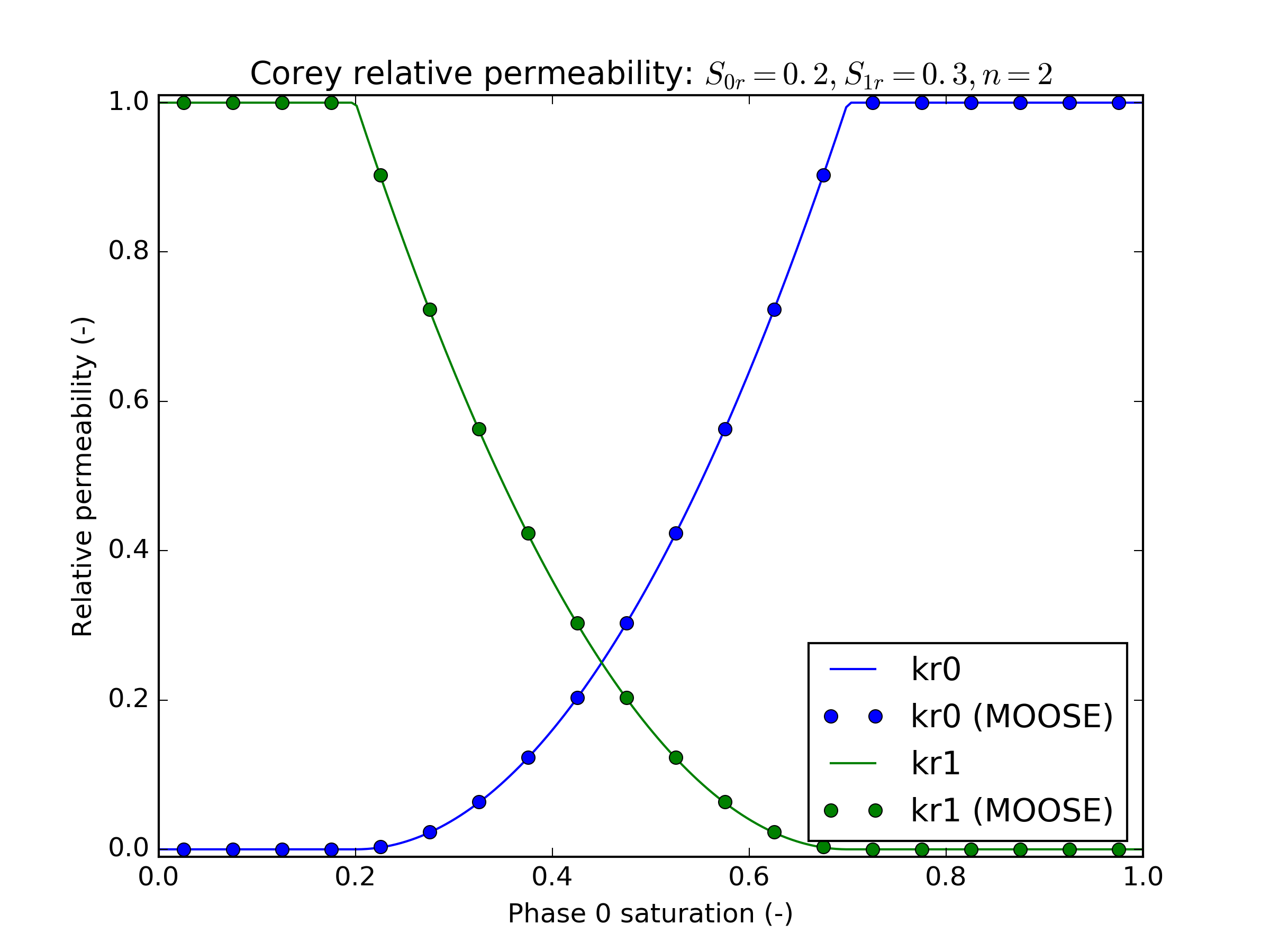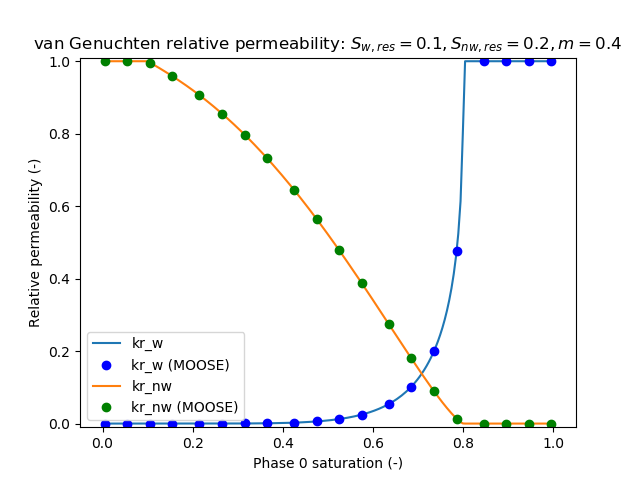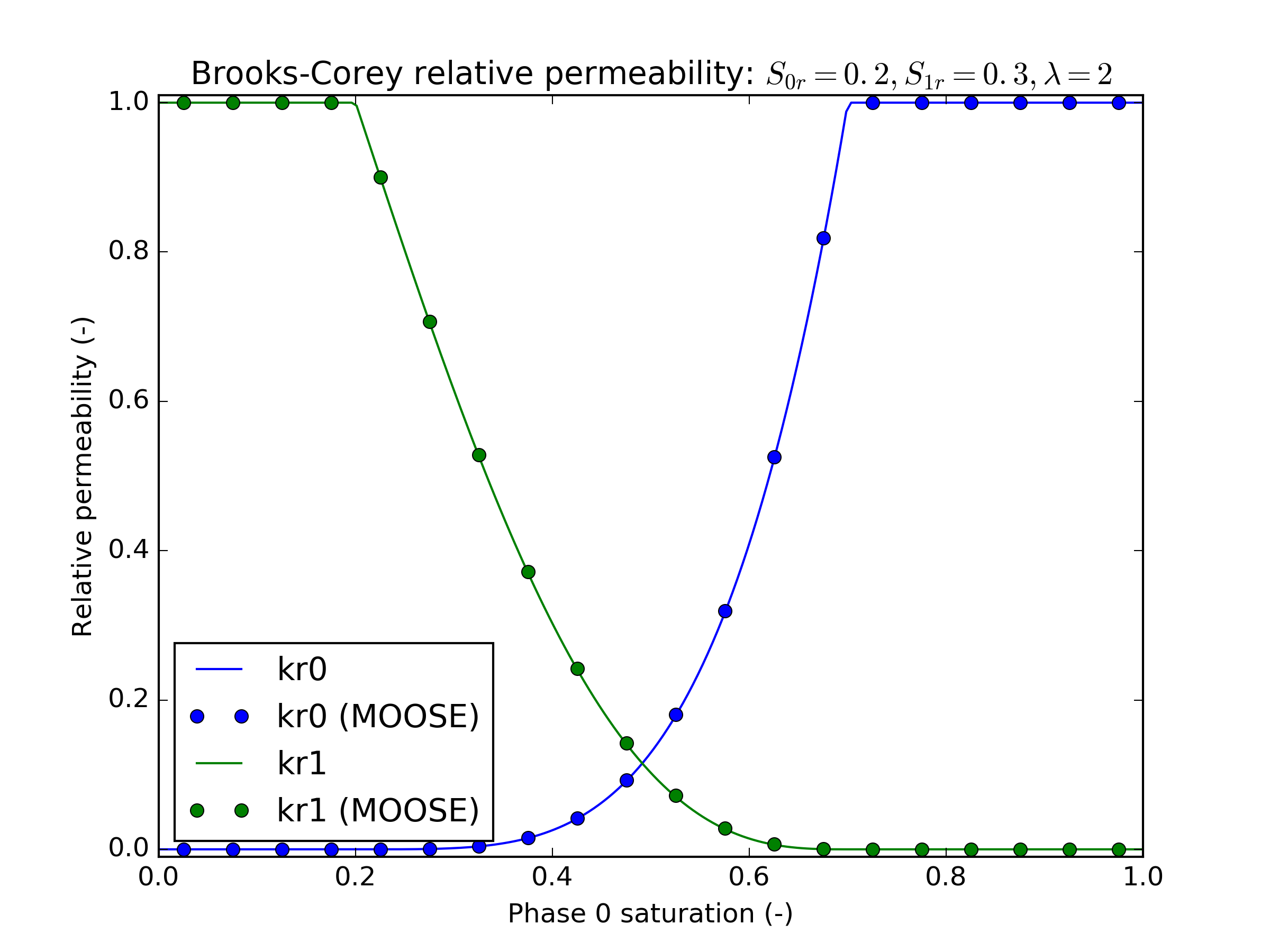# Relative permeability

The relative permeability of a phase is a function of its effective saturation: (1) In this equation:

• is the effective saturation for phase ;

• is the saturation for phase ;

• is the residual saturation for phase .

In the MOOSE input file, is termed s_res, and the entire sum is termed sum_s_res. MOOSE deliberately does not check that sum_s_res equals s_res, so that you may choose sum_s_res independently of your s_res quantities.

If then the relative permeability is zero, while if then the relative permeability is unity. Otherwise, the relative permeability is given by the expressions below.

## Constant

PorousFlowRelativePermeabilityConst

The relative permeability of the phase is constant (2) This is not recommended because there is nothing to discourage phase disappearance, which manifests itself in poor convergence. Usually as is a much better choice. However, it is included as it is useful for testing purposes.

## Corey

PorousFlowRelativePermeabilityCorey

The relative permeability of the phase is given by Corey (1954) (3) where is a user-defined quantity. Originally, Corey (1954) used for the wetting phase, but the PorousFlow module allows an arbitrary exponent to be used.Figure 1: Corey relative permeability

## van Genuchten

PorousFlowRelativePermeabilityVG

The relative permeability of the wetting phase is given by Genuchten (1980) (4) This has the numerical disadvantage that its derivative as tends to infinity. This means that simulations where the saturation oscillates around do not converge well.

Therefore, a cut version of the van Genuchten expression is also offered, which is almost definitely indistinguishable experimentally from the original expression: (5) Here the cubic is chosen so that its value and derivative match the van Genuchten expression at , and so that it is unity at .

For the non-wetting phase, the van-Genuchten expression is (6) As always in this page, here is the effective saturation of the appropriate phase, which is the non-wetting phase in this case.Figure 2: van Genuchten relative permeability

## Brooks-Corey

PorousFlowRelativePermeabilityBC

The Brooks and Corey (1966) relative permeability model is an extension of the previous Corey (1954) formulation where the relative permeability of the wetting phase is given by (7) and the relative permeability of the non-wetting phase is (8) where is a user-defined exponent. When , this formulation reduces to the original Corey (1954) form.Figure 3: Brooks-Corey relative permeability

## Broadbridge-White

PorousFlowRelativePermeabilityBW

The relative permeability of a phase given by Broadbridge and White (1988) is (9)

## FLAC

PorousFlowRelativePermeabilityFLAC

A form of relative permeability used in FLAC, where (10) This has the distinct advantage over the Corey formulation that its derivative is continuous at .

1. P. Broadbridge and I. White. Constant rate rainfall infiltration: a versatile nonlinear model, 1. analytical solution. Water Resources Research, 24:145–154, 1988.[BibTeX]
2. R. H. Brooks and A. T. Corey. Properties of porous media affecting fluid flow. J. Irrig. Drain. Div., 92:61–88, 1966.[BibTeX]
3. A. T. Corey. The interrelation between gas and oil relative permeabilities. Prod. Month., 19:38–41, 1954.[BibTeX]
4. M. Th. van Genuchten. A closed for equation for predicting the hydraulic conductivity of unsaturated soils. Soil Sci. Soc., 44:892–898, 1980.[BibTeX]